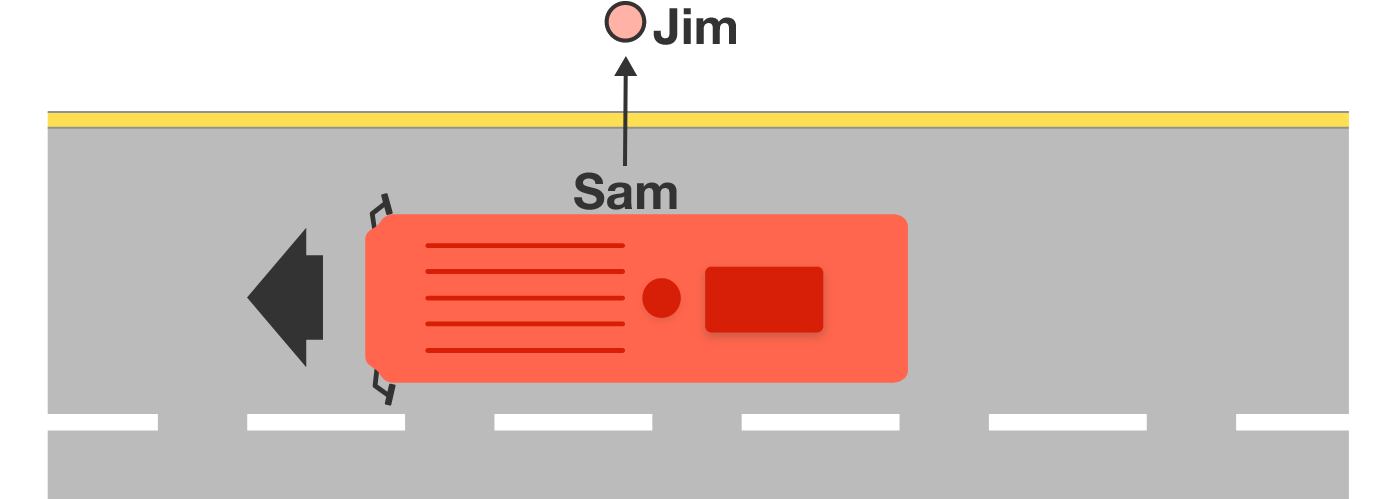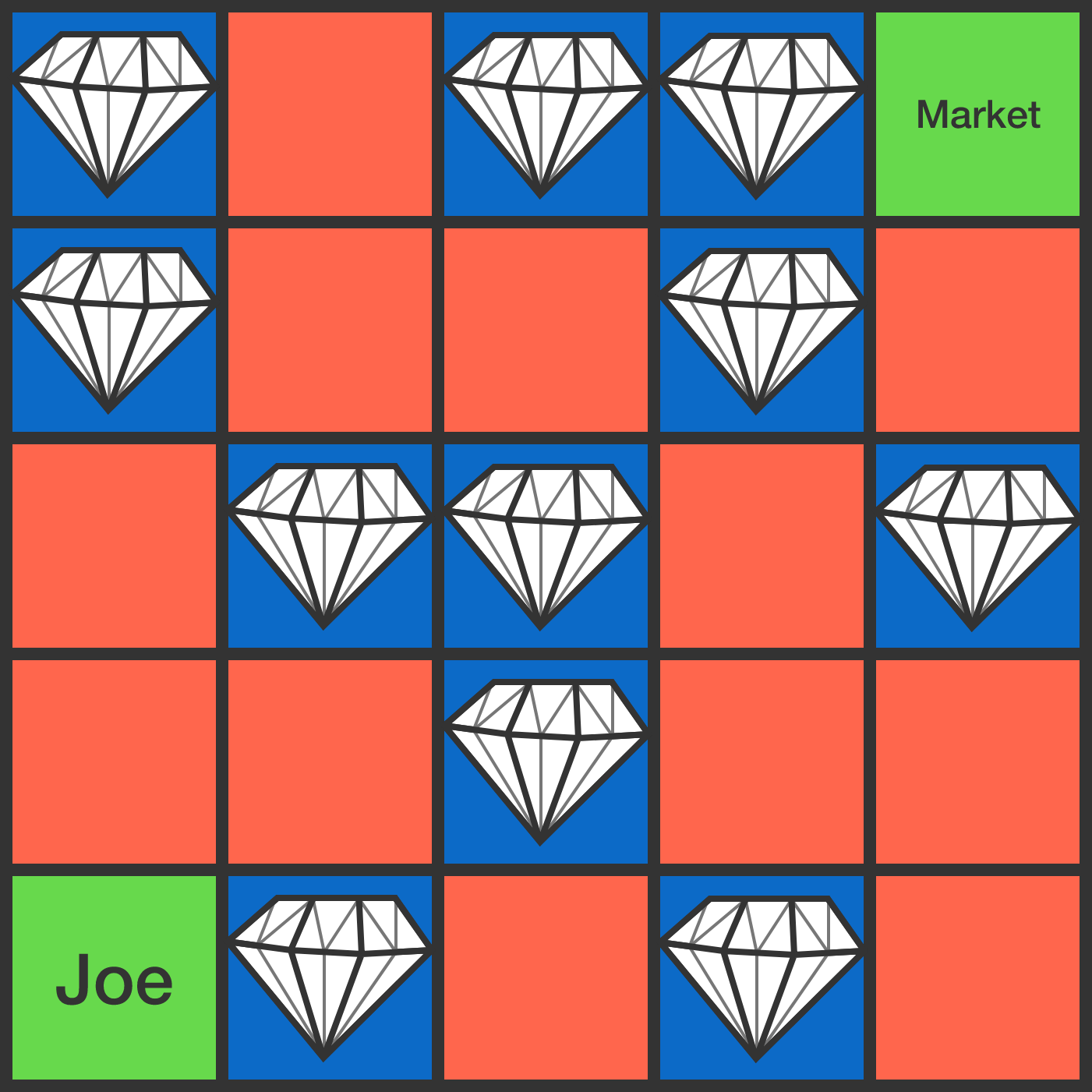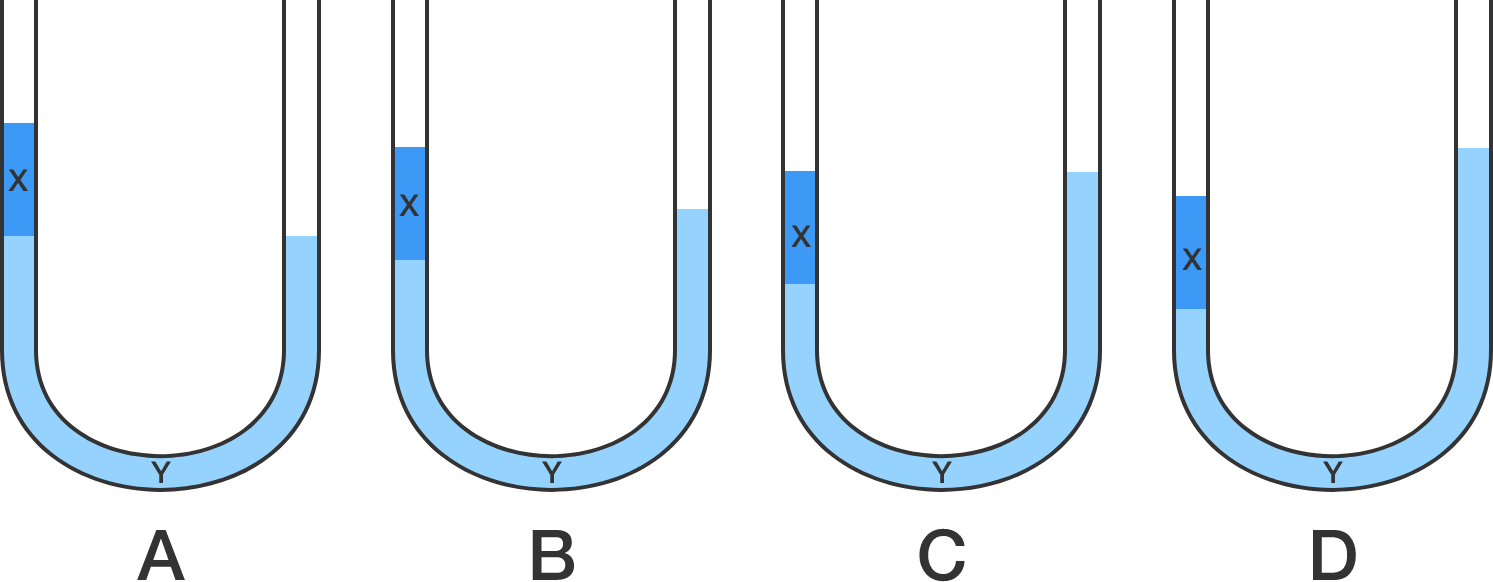# Problems of the Week

Contribute a problem

# 2017-08-21 BasicMischievous Sam is sitting in a moving bus. At the moment he spots his best friend Jim standing on the footpath, Sam throws a water balloon directly at Jim.

Will the balloon hit Jim?


Note: For simplicity, treat Jim and the balloon as if they are point particles.Joe (bottom left) is on his way to the market (top right), and he can only move up or right in each step.

In every cell he visits, there is either a gem or a guard. Each guard charges 1 gem to let him move on.

What is the maximum (net) number of gems Joe can gain along the way to the market?

Joe pours liquid $X$ (in dark blue) into the left arm of a U-tube that already contains liquid $Y$ (in light blue). The density of liquid $X$ is less than that of liquid $Y,$ and the two liquids are immiscible.

Which of the following represents the correct situation for the two liquids in the U-tube?$\begin{array}{ccccc} & & & \square & \square &\square \\ & & & \square & \square &\square \\ + & & & \square & \square&\square\\ \hline & & 2 & 5 & 5&5 \end{array}$

The above is a long addition with some numbers hidden in the boxes. Each box represents a distinct digit from 0 to 9.

Fill up all the boxes and find the digit not used.

\displaystyle \begin{aligned} \color{#3D99F6} 2 & \Longrightarrow {\color{#3D99F6} 2}{\color{#D61F06}2} = 2 \times 11 \\ \color{#3D99F6} 67 & \Longrightarrow {\color{#3D99F6}67}{\color{#D61F06}76} = 2 \times 3388 \\ \color{#3D99F6} 479 & \Longrightarrow {\color{#3D99F6}{479}}{\color{#D61F06}{974}} = 2 \times 239987 \\ \color{#3D99F6} 8123 & \Longrightarrow {\color{#3D99F6}{8123}}{\color{#D61F06}{3218}} = 2 \times 40616609 \\ \color{#3D99F6} 56209 & \Longrightarrow {\color{#3D99F6}{56209}}{\color{#D61F06}{90265}} = 5 \times 1124198053 \\ \color{#3D99F6} 999007 & \Longrightarrow {\color{#3D99F6}{999007}}{\color{#D61F06}{700999}} = 7 \times 142715385857 \end{aligned}

You have a machine that does some very interesting prime arithmetic. The machine takes in a prime number, reverses its digits, and attaches the resulting number to the prime input to form a new number.

It seems that for every prime number shown above (in the far left column), the machine produces a composite number (as implied by the right side of the equation).

Does there exist a prime for which the machine will produce another prime?

×